Disclaimer: This is an example of a student written essay.

Any opinions, findings, conclusions or recommendations expressed in this material are those of the authors and do not necessarily reflect the views of UKEssays.com.

# The effect of propagation and environmental factors on signal quality

Info: 3231 words (13 pages) Essay
Published: 13th Apr 2021

Development of a Resilient Wireless Sensor Network for Real-Time Outdoor Applications

Abstract

Wireless sensor networks that are deployed for any outdoor applications face the challenge of link variations. The outdoor sensor network would be affected because of unpredictable changes in the environment. The effect can vary accordingly with a single hop and a multi hop sensor network. In this paper, we analyze the causes of poor link quality, received signal strength and packet reception rate due to factors such as shadowing, fading, foliage, and rainfall. We use the packet-oriented simulation tool, Qualnet 5.0.2 to create a simulation framework. This enables us to observe the effects of the signal quality separately and independently after the environmental factors. The results developed from the simulation are tested and assessed with the data received from the real time wireless sensor network to monitor landslides located at Munnar, India.

## I. Introduction

Wireless sensor networks are extensively used for environmental monitoring, landslide detection, disaster management etc. These networks are mostly deployed in outdoor scenarios. These outdoor deployments of wireless sensor networks experience intermittent loss of link due to rainfall, foliage, fading and shadowing. This will affect the reliability of the network due to reduction in the packet reception rate. Hence outdoor wireless sensor networks needs an efficient fault tolerant mechanism capable to deal with the dynamic changes in the environment.

If you need assistance with writing your essay, our professional essay writing service is here to help!

For the design and development of a fault tolerant network it is highly necessary to accurately predict the expected dynamic changes in the environment and its effects on the network. The dynamic changes in an outdoor environment are due to rainfall rate, fog, vegetation, reflection, diffraction, shadowing etc. Due to these effects we cannot assure a line of sight path in every scenario. The non line of sight path causes the signal to degrade and cause the low quality in the transmission. To avoid the degradation of the link quality this work aims to determine the causes of link variation and the network parameters that get affected due to this change. Existing empirical models for rainfall, foliage, shadowing and fading are used to analyze the cause and effect relation using Qualnet software simulator. These analysis results will be used for developing an adaptive routing protocol that will increase the reliability of the system.

To find out how the environmental factors are affecting the signal quality a simulation model was done in Qualnet. By integrating the models in the Qualnet simulator the impact of different rate of packet transmissions were analyzed with respect to path loss and packet reception rate variations. It is observed that rainfall rate and propagation distance through foliage above a particular value affects the signal quality drastically than any other propagational parameters.

To investigate the accuracy of the simulated model, data from the real-time wireless sensor network system for landslide monitoring, deployed at Munnar, India was correlated with the simulated result and it showed about 95% similarity.

In this paper section II describes the related works. The propagational challenges prone to the wireless sensor networks are described in section III. The software architecture design used for the simulation is shown in section IV. The simulation results with various cases were shown in section V. Section VI conveys the conclusion and future works.

## II. Related Works:

A statistical analyzing is done. The linearity relationship between the received signal strength and link quality is calculated in Ekka  et al. In Nose et al. a signal strength based route construction is done to tolerate fault in the network. In most of the existing works the network performance was discussed either through any propagation effects, a combined analyzing is not done so far. This paper analyzed the combined effect of the propagational effects in the network quality.

## III. Propagational challenges:

The wireless sensor networks deployed in the outdoor can be affected by various propagational challenges such as path loss, fading, shadowing etc. Most of these propagational effects are mainly due to the environmental factors such as rainfall, foliage, fog, wind etc.

The effects of fading, shadowing, path loss, rain and foliage in WSN are studied in this work that are discussed in below sections.

### A. Foliage model selection:

Most of the empirical foliage loss models for the propagation path are exponential decay models, such as Weissberger model ,ITU Recommendation(ITU-R) model COST235 model, ITU-R model, Maximum attenuation (MA) model, Nonzero gradient (NZG) model, and Dual Gradient (DG) model .

In general, the exponential decay model has the following form :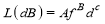(1)

Where A, B, and C are the parameters from different experiments with regression techniques.

The gradient models, the NZG model  was proposed by Seville to rectify the zero gradient problem associated with the MA model . However the NZG and MA model are not taking the frequency information as inputs. Hence by these models, we cannot analyze the propagation effects of different frequencies. Subsequently, the DG model is proposed with the antenna beam width and the operating frequency as the input parameters. since there is no frequency information in both the NZG model and MA model .

The different models based on horizontal path propagation with its empirical formula are as follows:

Weissberger model :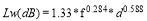(2)

Where f is the frequency(GHz) , and d is the distance of propagation through foliage it should be between 14 meter and 400 meter.

ITU-R model :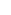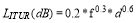(3)

Where f is the frequency in MHz, and d is the tree depth in meter.

MA model :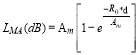(4)

Where Am is the maximum attenuation, R0 is the initial gradient of the attenuation rate curve, and d is the distance of propagation through the foliage.

Since most of the wireless sensor networks uses GHz frequency range for communication, the weissberger model for determining the path loss effects in WSN.

### B. Rainfall model:

The rainfall model is used to study attenuation in the transmited signal due to rain fall. Many scattering models are existing to find the signal degradation due to rainfall. But all those scattering models require the complex calculation of the distance between the scattering object and the receiver and the transmitter.

The rainfall attenuation model’s applied equation is :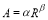(5)

Where, R is the rainfall rate in millimeter per hour.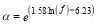(6)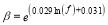(7)

Rayleigh fading distribution is as follows :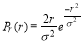(8)

Where r is the rms value of the voltage of the signal and sigma is the standard deviation from the expected mean value.

### D. Log Normal Shadowing Model:

The large scale signal power strength model is used for predicting the average signal strength as a function of distance between the Transmitter and Receiver which may include antenna gains, height, and frequency of operation. The path loss model does not discriminate between two locations which are at the same distance from the base station, but are at two distinct directions. This is due to the fact that the path loss model is not considering the effect of local clutter. In reality if we consider two locations then the local mean of the path losses will vary. The Path loss model only conveys an average value of path loss of the transmitted signal in a region or area. The local mean is a random value and its effect is calculated through the shadowing model. Thus the Path loss formula is extended in order to taken care the local mean variation as well. Hence the combined effect of the path loss and the shadowing are considered to calculate the received signal strength at a distance‘d’ :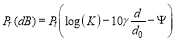(9)

Where:

K is a constant which depends on the antenna characteristics.

ð›¾ is the path loss exponent.

ð›¹ is the Gaussian distributed random variable.

The above mentioned propagation models are used to analyze the characteristics of the degrading signal such as received signal strength and the packet loss due to poor link quality.

## IV. Software Architecture:

The architecture is designed in such a way to study the characteristics of the output signal with the different effects of the propagational parameters. In the figure 1, the propagation models module includes the fading model, shadowing model, rainfall attenuation model and vegetation attenuation model.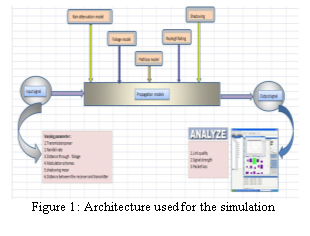Fig 1: Architecture used for the simulation

The output signal is analyzed with link quality, received signal strength and the packet reception rate. The link quality is the ratio in the received signal strength to the noise power. Signal strength is the received signal strength received at the receiver.

Packet loss is the number of packets received out of the total packet sent. It can also be inferred as the packet reception rate.

## V. Simulation and Results:

#### A. Simulation modeling for rainfall attenuation model:

According to equations (6), (7) and (8) we created different simulation scenarios in Qualnet to obtain a series of data with the link quality and received signal strength .The simulation results obtained using Qualnet GUI interface are shown in figure 5 and figure 6.

Our academic experts are ready and waiting to assist with any writing project you may have. From simple essay plans, through to full dissertations, you can guarantee we have a service perfectly matched to your needs.

#### B. Simulation of path loss:

In Qualnet simulator a sensor network scenario is created where the sender node is sending a total of 100 packets. This scenario is used to infer the effect of path loss in the transmitted signal with combined effect of the environmental parameters such as the rainfall rate and the distance of propagation through foliage. Then at different transmitter-receiver distance, the path loss is calculated and plotted the graph in Matlab.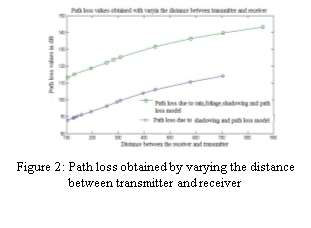From the investigation of the graph shown in figure 2, a 40 dB difference in the path loss estimated without the effect of the environmental factors than with the effect of the environmental factors.

#### C. Simulation of packet reception rate:

The packet reception rate is analyzed with the combined effect of the environmental factors like the rainfall rate and the distance of propagation through foliage. The result in figure 3 shows the packet loss due to the effect of environmental factors is higher than the scenario where there is no effect of environmental factors. This is due to the rainfall attenuation and the foliage effect. Analyzing the effects of these two factors is needed drastically to find out the minimum level of rainfall rate and the distance of propagation through foliage which affects the degradation of the signal.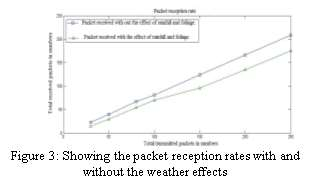On analyzing the graph it conveys that rainfall

Rainfall rate above 350 millimeter per hour is

Environmental factors increase the effect of path loss which results in the reduction of the link quality.

#### D. Effect of shadowing mean in the transmitted signal:

The shadowing mean is varied and analyzed the packet loss with the variation.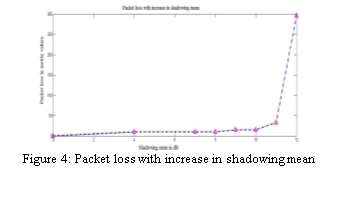From the analysis of the simulation result, it is clear that the packet loss started when the shadowing mean is above 4dB. All the packets are lost when the shadowing mean is above 11dB.

#### E. Effect of Rainfall rate in the transmitted signal:

The variation in the rain fall rate has affected the signal only above 350mm/hr. No packet loss is observed within the range of 50-350mm/hr .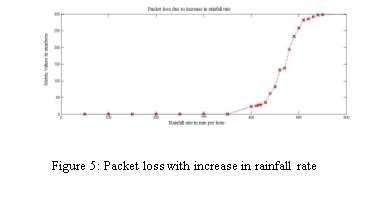The reduction in the link quality can result in packet loss is proved through simulation result which is shown in figure 6.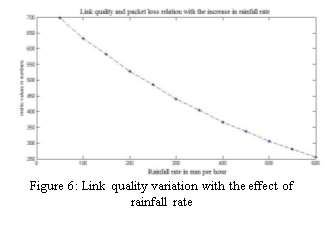Also the link quality of the signal is analyzed and

The link quality is observed to be decreasing with increase in rainfall rate.

#### F. Effect of foliage in the transmitted signal:

The distance of propagation through the foliage, affects the signal quality. Even the movement of vegetation due to degrade the signal quality which is not investigated in this paper.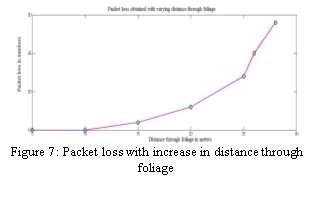In figure 7, the simulation result of foliage model is shown. If the distance through foliage is more than 10 meters, it will affect the packet reception rate. The link quality is also analyzed with the variation in the distance through foliage.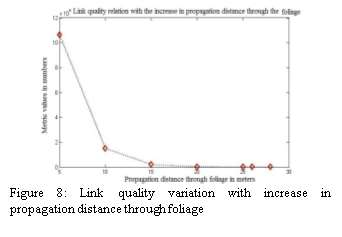The result from graph in figure 8 shows if the distance through propagation is above 10 meters can affect the signal quality.

#### G. Effect of transmission power in the packet loss:

With the result obtained using the different rainfall rates which is shown in figure 9, it is clear that when rainfall rate is above 5.833 mm per minute packet loss is observed. So assuming the rainfall rate to be 5.833 mm per minute the distance through foliage is varied and the result is analyzed.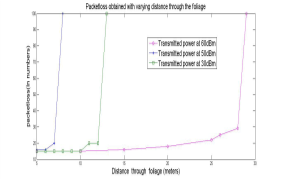Figure 9: Varying the distance through foliage with different transmission powers

#### H. Real Data Analysis:

The real data is received from the real-time wireless sensor network system for landslide monitoring, deployed at Munnar, India. 90% match with simulated result and real data from munnar is obtained in the real data analysis result shown in figure 10.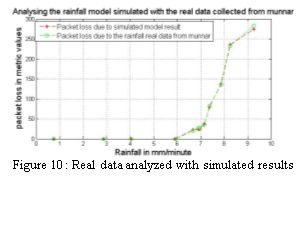The real data from landslide monitoring system is analyzed with the simulated result in the Qualnet with the combined effect of the environmental parameters like the rainfall rate, foliage, shadowing and fading. The result holds the fact that the models were showing similar results as in theoretical models.

## VI. Conclusion and Future works:

To analyze the effect of propagation and environmental factors on the signal quality we implemented simulation of these models in the Qualnet. Simulation result shows the rainfall rate and the distance of propagation through foliage have a major effect on the performance of the network especially on the link quality and packet reception rate. Then the simulated result is analyzed with real time wireless sensor network system for landslide monitoring deployed at munnar.

In future from these analyzed results we are planning to design an adaptive routing protocol that adapts its path with the best available link quality.

View all

## DMCA / Removal Request

If you are the original writer of this essay and no longer wish to have your work published on UKEssays.com then please:

Related Services

Related Lectures